APPLIED INDUSTRIAL CONTROL SOLUTIONS LLCPAPER No. 4

The Effect of Speed Loop Bandwidths and Line-speed on System Natural Frequencies in Multi-Span Strip Processing Systems

1997 IEEE IAS Annual Meeting

Brian Thomas Boulter
Applied Industrial Control Solutions LLC
4597 E Sprague Rd.
Independence, OH, USA 44131

Abstract - Web Handling and Strip Processing systems (SPS’s) are composed of multiple tension zones. These zones are separated by driven rolls such as bridles whose speed is regulated by a closed loop controller. Given that the typical tension regulator regulates tension by trimming the reference to a closed speed loop controller, the designer of the tension regulator cannot ignore the effects of closing the speed loop, and line speed, on the strip processing system natural frequencies. These natural frequencies are typically computed as the eigenvalues of an equivalent translational cascaded spring-mass system. This paper discusses these effects.

I. INTRODUCTION

Most frequency domain analysis of strip processing systems (SPS's) involves obtaining the transfer functions from an input variable of interest to an output variable of interest. The most common transfer functions used in this type of analysis are those that provide the analyst with spectral information about system variables that directly affect product quality, such as strip strain (or tension) and roller velocity. For example, the transfer function from shaft torque to tension feedback, or from shaft torque to speed feedback are of interest to both the control system engineer and the O.E.M. The control system engineer is interested in these transfer functions because he is responsible for designing the speed and tension regulators and these variables are controlled through the shaft torque produced by the motor. The O.E.M., because he is interested in ensuring the control system vendor satisfies performance guarantees on speed and tension regulation that directly affect the quality of the final product.

System eigenvalues (or natural frequencies) are defined as the roots of the characteristic polynomial of a system of linear time-invariant (LTI) differential equations. In a linear analysis of the SPS the denominator of all SPS transfer functions will be composed of poles whose location in the s-plane are equivalent to the system eigenvalues. Often the analyst assumes the SPS can be modeled analogous to a mass-spring system where the roll inertias (Ji) and the strip springs (Ei*Ai /Li) are analogous with the translational masses (Mi) and springs (ki). The transfer functions from torque (ti) to speed (wi) and tension (Tj) are assumed to be analogous to the transfer functions from a disturbance force (fi) acting on the mass (Mi) to the translational speed of the mass (Vi) and the force in the spring {ki(xi-xi-1)}. This approach does not lump the closed speed loop regulators into the plant model. In a more practical sense, the eigenvalues resulting from such an analysis can be defined as the poles of the transfer function from any motor torque ti to any tension feedback Tj, or speed feedback wi, in a SPS where all the speed regulators are operating open loop.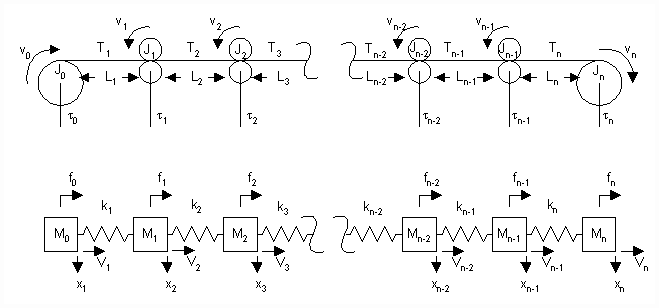Figure 1. A Typical Mass-Spring SPS Representation

Figure 1 shows the rotational system and the equivalent mass-spring translational system from which the eigenvalues of such an analysis are typically obtained. It is composed of an entry section fed by a winder that unwinds unprocessed strip, a process section where proprietary strip processing is performed, and a delivery section that winds the processed strip into rolls.

Figure 2. Is a single line diagram of a typical SPS including the drives and speed loops. The entire system may be divided into 'n' tension zones that are separated by 'n+1' driven rolls. The unwinder (payoff reel), winder (tension reel) and driven rolls (bridles, calenders etc.) are in turn driven by a power source (Current/Torque Minor Loop - CMLi). The reference to the CMLi is proportional to a desired torque (ti) and comes from a speed regulator (a PI regulator - SPIi) that regulates speed to match a desired speed reference wri.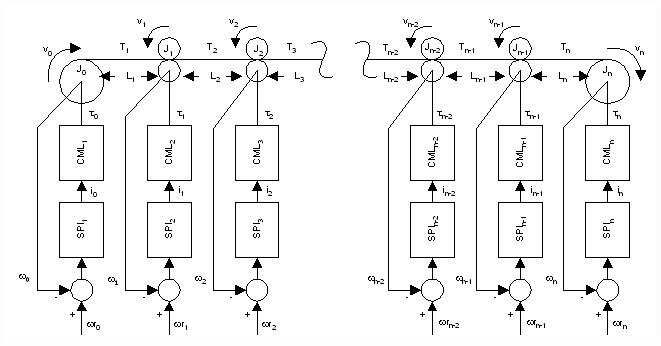Figure 2. A Typical SPS Including Drives and Speed Loops.

Given that tension regulation in strip processing systems is performed by an outer tension major loop that cascades into a speed minor loop, the eigenvalues of SPS’s that are obtained without the inclusion of the closed speed loops are not useful to the control system engineer responsible for the design of SPS tension regulators. Rather, the design of a single-input, single-output (SISO) tension regulator for the 'ith' section in Figure 2. will require an approximation of the transfer function from the speed reference (wri) to the tension feedback (Ti or Ti+1). This transfer function, which includes the closed speed loop, represents the plant the tension regulator is controlling. The tension regulator may use the tension feedback Ti (i.e. drawing material to control the tension in the 'ith' section) or Ti+1 (feeding material to control tension in the 'i+1' section) to regulate the tension around a desired setpoint.

There are exceptions to the cascaded architecture. Specifically, in winders, a direct reference to the CML from either a tension or current regulator may be used. However for the sake of brevity only the cascaded architecture will be discussed in this paper.

Obtaining a transfer function from wri to Ti is a fairly simple task if there are only two masses and one strip section (e.g. an unwinder and a winder directly coupled by the strip). Approximate transfer functions for this application are derived in , . However the coupling of additional sections into the system makes the derivation of an analytically exact transfer function unwieldy and, as sections are added, beyond the capabilities of even the most sophisticated symbolic math programs.

While the approach taken in this paper is one based on linear system theory, the author avoided presenting cumbersome sets of transfer functions and their derivations. This was done with the knowledge that, in the analysis of an actual strip process application, there exists a profusion of non-linear contributions that render exact linear transfer functions more or less inaccurate, depending on the application. The approach taken in this paper is one that describes the expected behavior of the transfer functions in terms of the effect of speed loop bandwidths, and line speed, on the system eigenvalues. The expected behavior is expressed as a series of observations phrased in terms of closed speed loop bandwidths, line speed time constants, and natural frequencies.

II. ANALYSIS

1. Nomenclature

 JMOTOR motor inertia [kg m^2] JLOAD, reflected roll (load) inertia [kg m^2] JI JMOTOR + JLOAD [kg m^2] Vi i’th roll surface velocity [m/min] wi i'th motor rotational velocity feedback [rpm] wri i'th motor rotational velocity reference [rpm] qi i'th motor shaft position [rad] w(CML)i i'th CML bandwidth [rad/sec] wS,i i'th speed loop PI lead freq. [rad/sec] wCO,i i'th speed loop crossover [rad/sec] KSi i'th speed loop PI prop. gain KSHAFT spring constant of the drive shaft [kg m^2/rad] Ri i’th roll radius [m] Di i’th roll diameter [m] GRI i’th roll gear ratio Li i’th tension zone length [m] Ti i’th tension zone tension [kgf] ti i’th roll reflected shaft torque [kgf m] tMAX i’th motor maximum torque [kgf m] iI instantaneous CML current [A]. iMAX maximum CML current. [A] E modulus of elasticity [kgf/mm^2] A cross sectional area [mm^2] S Laplace operator LS maximum line speed [m/min] LSI operating line speed [m/min]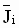i’th per-normal inertia (see Eq. 4) KI strip-spring constant (see Eq. 8) tV strip-span velocity time-constant (see Eq. 9) Wi span natural frequency [rad/sec] (see Eq. 12)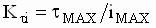torque loop gain [kgf m/A]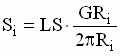i'th motor gear-in speed [rpm]

B. Plant Representation

The strip tension equation (1) is a commonly used equation (, , ) describing the dynamics associated with the conveyance of strip through tension zones. It is based on the principle of conservation of mass in a mass-flow system and is derived in ,.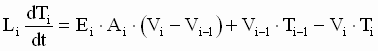; (1)

where: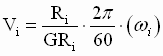(2)

The motor/load torque equation is given as: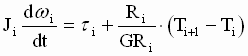; (3)

In Figure 3. an s-domain block diagram of equations (1-3) is presented. It includes the hooks that allow coupling multiple sections together, it does not include any damping terms. Note that a rigorous representation of equations (1-3) requires the integrators in Figure 3 to be preset to their respective initial conditions.

For a given operating line velocity LSi, an approximate linear representation of equations (1-3) can be obtained , . A block diagram of the linearized model is shown in Figure 4.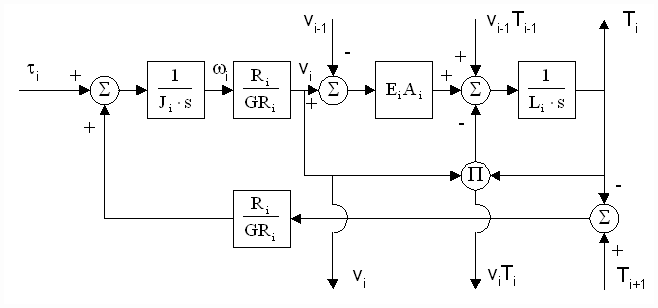Figure 3. Block Diagram of a Strip Tension Zone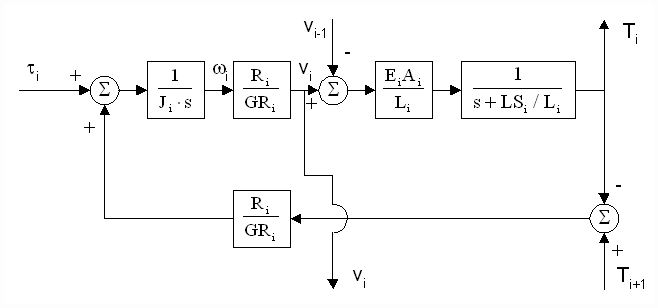Figure 4. Linearized Block Diagram of a Strip Tension Zone

A linear s-domain block diagram approximation of the plant (Figure 5), as seen by a cascaded tension loop regulator, can be obtained by closing a speed loop around the speed feedback wi in Figure 4.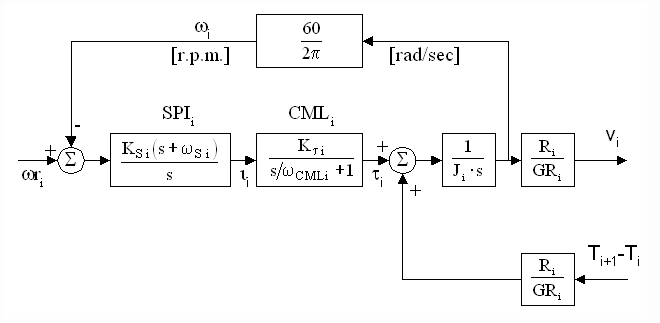Figure 5. Plant Model Including the Speed Loop

Cascading n sections of the model in Figures 4 & 5 provides a plant model from which an investigation of the effects of speed loop bandwidths on system eigenvalues can be conducted.

C. Some Usefull Quantities

To simplify the analysis of the multi-span strip processing system the following set of quantities are most useful.

Per-Normal Inertia

Let the per-normal inertia of a system be defined as the time it takes [sec] to accelerate the motor and load inertia to the application gear-in speed (Si) with maximum motor torque (tMAX) It is defined as: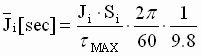(4)

Speed Loop Bandwidth

The bandwidth of a closed speed loop is limited to the frequency at which the magnitude of the speed feedback in response to a sinusoidal input is attenuated 3[db] from the setpoint magnitude. It is also approximated as the crossover of the open speed loop Bode plot. For the speed loop shown in Figure 5. it can be shown that the open loop crossover and hence the approximate bandwidth of the speed loop is: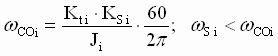(5)

In any drive system the bandwidth of the speed loop will be constrained by the mechanical integrity of the drive train. As a general rule the bandwidth must be an order of magnitude lower than the lowest torsional frequency in the drive train. This constraint can typically be satisfied by: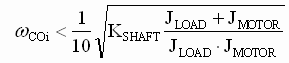(6)

In addition, to avoid torque jitter in the motor shaft, the per-normal controller proportional gain should be limited to 60. For industrial motors, this constraint can be accommodated by limiting the bandwidth to: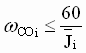(7)

Strip Spring Constant

Let the strip spring constant be equal to: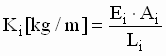(8)

Strip-Span Velocity Time-Constant

Let the strip-span velocity time constant be equal to: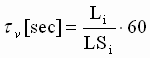(9)

Normal Eigenvalues

The simple mass-spring system (Figure 1) can be defined in rotational terms as a set of differential equations expressed in the Laplace domain (10):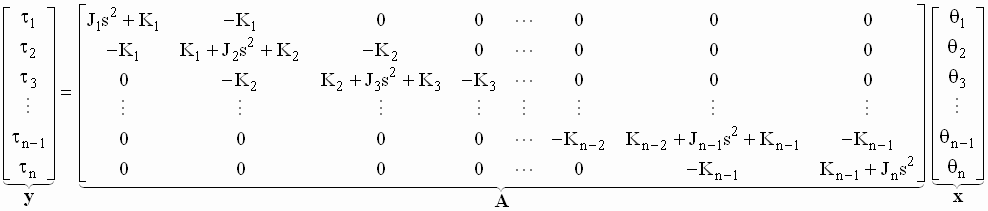(10)

Let the normal eigenvalues be defined as the roots of the determinant of A.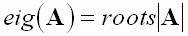(11)

Span Natural Frequency:

Let the span natural frequency be a measure of the frequency at which the strip span spring (Ki) and attached inertia (Ji) would exchange energy if the strip spring was terminated at an infinitely large mass.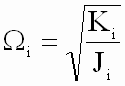(12)

D. Observations

Following is a series of observations that are intended to provide the reader with an intuitive understanding of the effect of speed loop bandwidths and line speeds on SPS system eigenvalues (or transfer function poles).

Analysis of a 9 section - 10 inertia SPS provides supporting bode plots for each observation. The SPS is comprised of sections structured the same as shown in Figures 4 and 5. Each Observation is followed by a brief discussion, and a plant description for the particular set of Bode plots presented. The plant description is in terms of the quantities described in section 2.3.

The short discussion is provided to clarify the observation in terms of the stated effect on the poles of the transfer functions (or the system eigenvalues) from speed reference wri to tension feedback Ti and speed feedback wi. The discussions are intended to be of practical use to the control system engineer responsible for the design of SPS tension regulators.

In addition, it is assumed that the bandwidth of each CML is high enough that in the frequency range of interest it behaves as a linear gain element. Its dynamics can, therefore, be ignored.

Observation No.1

As additional driven sections are added to a SPS the magnitude of all existing eigenvalues will decrease.

Discussion: Consider a multi-span SPS without speed regulators, the denominator of the transfer function from any ti to any tension feedback Ti will be composed of poles that can be factored into real poles or complex pole pairs. If a section is added to the SPS the magnitudes of all the existing poles and complex pole pairs in the transfer function from any ti to any tension feedback Ti will decrease.

This implies that the longer a SPS process line is, the lower the eigenvalues will become. A good way to visualize this is to create a purely symmetric SPS and observe the effect of adding symmetric sections to the SPS on the Bode plot of the transfer function from a torque tn/2+1/2 to a tension feedback Tn/2+1/2 in a SPS with ‘n’ sections. A symmetric SPS is one that has been designed so that the strip span natural frequencies are all approximately the same. Similarly, a symmetric SPS will have sections with very similar per-normal inertias. Figure 6 is a collage of 3 Bode magnitude plots of the transfer function of tn/2+1/2 to a tension feedback Tn/2+1/2. The number of sections varies from five to nine in increments of two.

Plant Description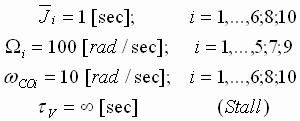In Figure 6, the solid line is from the analysis of the 9 section SPS, the dotted line: the 7 section SPS, and the dashed line: the 5 section SPS. It is clear that as symmetrical sections are added the magnitude of the eigenvalues decreases.

From the perspective of the tension regulator designer, the problematic plant poles in long SPS’s are significantly lower than the strip span natural frequencies calculated using (12). It is important to keep this perspective in mind when commisioning the tension regulators. In the authors experience the dominant problematic natural frequencies in SPS’s are typically lower than those calculated using linear analysis techniques.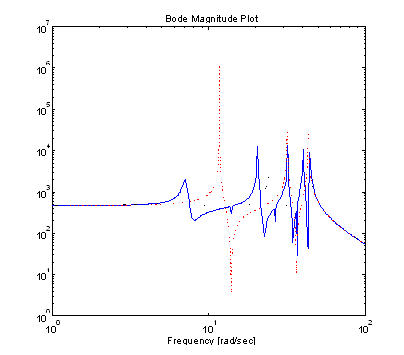Figure 6. Observation 1. Bode Magnitude Plot of tn/2+1/2 to Tn/2+1/2 for n=5,7, and 9 Section SPS’s

Observation No.2

2.a) If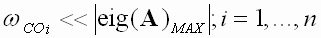the eigenvalues of a SPS system that includes the closed speed loops are approximately equal to eig(A).

Discussion: If the magnitude of all the SPS normal eigenvalues are much greater than the bandwidth of all of the speed loops, the poles of the transfer function from any wri to any closed speed feedback wi or any tension feedback Ti will be approximately the same as the poles in the transfer function from any torque ti to any to any speed feedback wi or any tension feedback Ti in a SPS with no speed loops.

2.b) If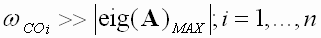the eigenvalues of a SPS system that includes the closed speed loops are approximately equal to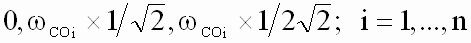Discussion: If the bandwidths of all the speed loops are significantly higher than the magnitude of the maximum normal eigenvalue, the denominator of the transfer function from any speed reference wri to any speed feedback wi or any tension feedback Ti will approximately consist of a pole at zero and two real poles at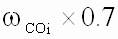and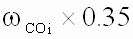2.c) The damping of all normal eigenvalues with magnitudes greater than the speed loop bandwidth for a given tension zone will increase as the ratio of the eigenvalue magnitude to the speed loop bandwidth decreases.

Discussion: Speed loops produce a damping effect on natural frequencies. The closer the natural frequency is to the speed loop crossover the more pronounced this damping effect will become.

To demonstrate the effects described in Observation No.2 a collage of 10 Bode plots (Figure 7.) of the transfer function from wr5 to T5 for the example SPS is presented. The per-normal inertias, speed loop crossovers and span natural frequencies for all 9 sections are identical in each plot. This results in a highly symmetrical plant. The 10 plots are obtained from an analysis performed with 10 logarithmically spaced span natural frequencies that vary from 6 to 600 [rad/sec]. For each plot the natural frequency of all 9 tension zones is identical.

Plant Description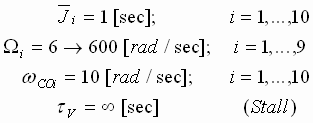Some additional observations from Figure 6:

1. The low frequency gain of the transfer function does not change significantly until the eigenvalues are low enough for the speed loop to provide damping. For a system that is highly symmetrical, the gain changes linearly with Wi when Observation 2.b is satisfied.
2. The speed loop PI lead frequency wSi provides the observed lead break frequency at 2 [rad/sec]. wSi effectively cancels the lower pole of the real pole pair that results when Observation 2.b is satisfied.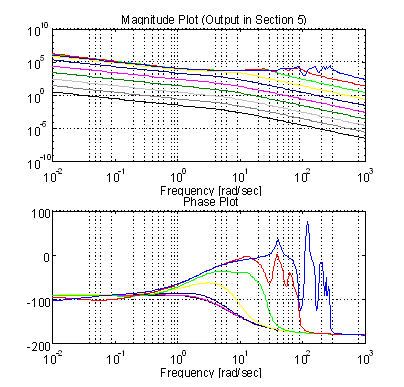Figure 7. Observation 2. Bode Plot of wr5 to T5

Observation No.3

Dominant speed loop damping effects result from the immediate speed regulator and the speed regulators acting on the preceding and following sections.

Discussion: Damping of the normal eigenvalues for a given tension zone is dominated by the bandwidth of the speed loop driving that section, the second most dominant factors are the speed loop bandwidths of the sections preceding and following the given tension zone.

To demonstrate this observation, consider a process line that is composed of two sets of high bandwidth speed regulators separated by a single low bandwidth speed regulator. Poor damping of the system eigenvalues will be observed around the section driven by the low bandwidth regulator. Figures 8,9,10 represent the transfer functions from wr3 to T3, wr4 to T4, wr5 to T5, respectively. The SPS has 10 [rad/sec] speed loop regulator bandwidths for sections 1,2,4-10. The bandwidth of Section 3 is set at 0.1 [rad/sec]. Note that there is little difference between Figure 10 and Figure 7. This indicates that the drive in section 4 provides section 5 with considerable isolation from section 3. As in Figure 7 Figures 8,9,10 are composed of a collage of 10 Bode plots resulting from an analysis of the SPS with 10 logarithmically spaced Wi’s that vary from 6 to 600 [rad/sec].

Plant Description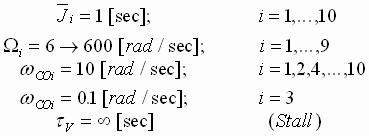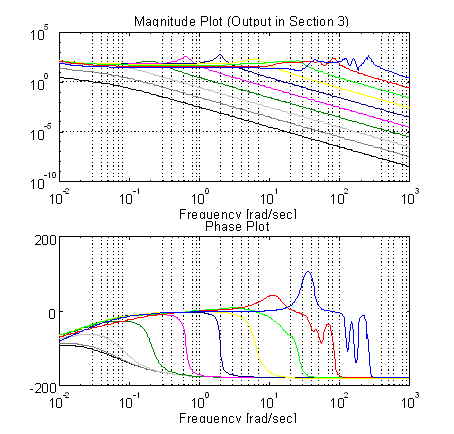Figure 8. Observation 3. Bode Plot of wr3 to T3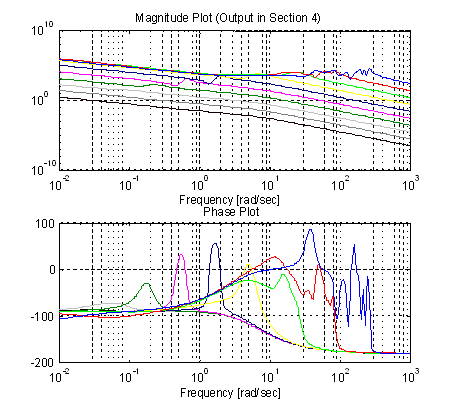Figure 9. Observation 3. Bode Plot of wr4 to T4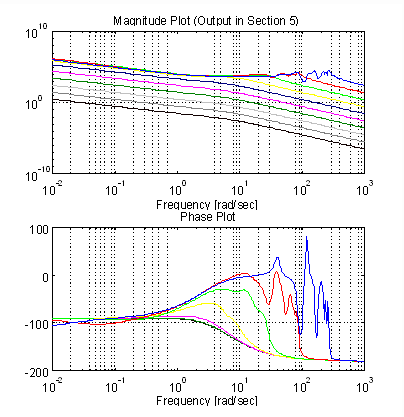Figure 10. Observation 3. Bode Plot of wr5 to T5

Observation No.4

Damping of the normal eigenvalues will increase with line speed.

Discussion: The damping of the normal eigenvalues is worst case at stall. When the strip begins moving there is a damping effect that is the result of the bulk flow of the material through the tension zone. As a result of the lack of damping at stall, it is not uncommon for drive system vendors to operate tension regulators as proportional only controllers at stall, switching to a PI configuration at a pre-determined strip line speed.

To demonstrate this a plant with a fixed a set of WI’s equal to 600 [rad/sec] is analyzed with a set of 10 logarithmically spaced velocity time constants tv varying from 10 to 0.01 [sec] (Figure 11).

Plant Description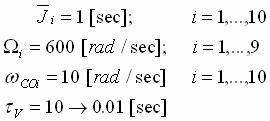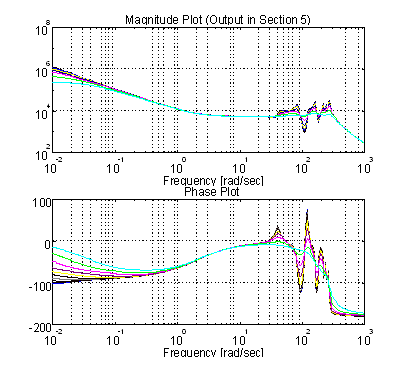Figure 11. Observation 4. Bode Plot of wr5 to T5

Observation No.5

The pure integration in the transfer function from wri to Ti at stall appears as a real pole that gravitates from the origin of the s-plane towards a value of -1/tV as bulk movement of the strip occurs. The higher the damping of the eigenvalues the better the approximation of -1/tV as the pole location.

Discussion: At stall, a pure integration is observed in the transfer function from wri to Ti. The moment the bulk movement of the strip begins, the integration becomes a real pole. For most systems this pole is very low in frequency and does not significantly alter the low frequency gain of the plant. When the damping of the normal eigenvalues is such that Observation 2.b is satisfied, the pole location can be approximated as -1/tV.

This phenomena can be problematic in certain tension regulation schemes. For example, assume a proportional tension regulator is used in a SPS with an extremely high top line speed of 3000 [m/min] and a length between driven sections of 10 [m]. In addition, assume that the speed regulators have ample bandwidth and satisfy Observation 2.b. The transfer function from wri to Ti in a system with such a line speed will result in a pole at 5 [rad/sec]. A significant loss in low frequency gain can be expected and a steady state error in the closed tension loop will be observed. To demonstrate this a plant with a fixed a set of Wi’s equal to 10 [rad/sec] is analyzed with a set of 10 logarithmically spaced velocity time constants varying from 10 to 0.01 [sec] (Figure 12).

The movement of this pole can also result in a type of low frequency instability in PI tension regulators. If the designed crossover of the tension loop is less than 1/tV, the loop will tend to "die" and loose its ability to regulate tension as the speed of the line increases past a certain threshold speed. The exact speed at which this will occur will vary depending on the speed loop bandwidth, the associated eigenvalue damping, and the phase margin built into the tension loop. This instability is not a true instability in the classical sense of the word, it is rather an observed drifting or wandering of the tension that can be attributed to a combination of integral action in the regulator, low tension loop bandwidth, and system noise.

Plant Description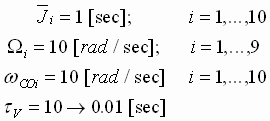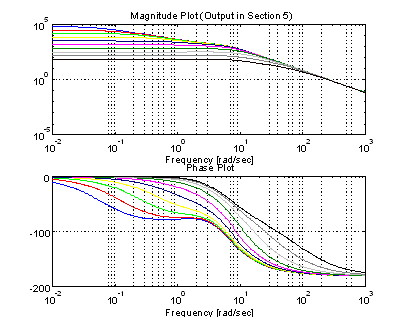Figure 12. Observation 5. Bode Plot of wr5 to T5

III. CONCLUSIONS

It is clear from the above discussion that the designer of cascaded tension regulators for multi-span strip processing systems should consider the effect of the speed loop bandwidths on the s-plane location of the SPS eigenvalues when designing tension regulators. Based on the above discussion several conclusions can be drawn:

A. The higher the bandwidth of the SPS speed regulators the greater the damping of problematic SPS natural frequencies.

B. If possible, the O.E.M. process design team should strive to create symmetric systems (similar Wi’s in all the sections and per-normal inertias that are approximately equal). This enables consistent damping of SPS eigenvalues with section speed regulators that have identical bandwidths.

C. The per-normal inertias (and by implication the motor horsepowers) and the torsional stiffness of the drive shafts should be selected such that the speed regulators can be tuned for wCO’s that will provide adequate damping of the expected SPS eigenvalues. This significantly improves the performance of the tension regulators.

D. O.E.M.’s should be aware that compensation as a function of line speed may be required in tension loops as line speeds increase and the span lengths decrease.

E. If an LTI linear system analysis is used to determine the SPS eigenvalues it can be considered a worst case analysis if the eigenvalues are computed to determine the minimum bandwidth of the speed regulators required to satisfy Observation 2.b. The actual eigenvalues of the system will always be less than or equal to these analytically derived values.

A. Tuning The Tension Regulator

As a note of interest, the tuning of a tension regulator for a SPS with speed loop bandwidths that satisfy Observation 2.b for all the product that is processed through the system becomes a fairly simple task. The lead and lag frequencies of a lead/lag compensator and a PI regulator lead are tuned as shown below: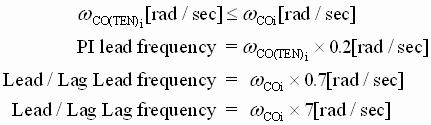Briefly, the PI lead frequency of the PI controller should be set to 1/5 the desired tension loop crossover. The tension loop crossover should be less than or equal to the speed loop crossover. The lead/lag lead frequency is set to approximately cancel the damped pole from the plant at 0.7 times the speed loop crossover. Recall that the pole at 0.35 times the speed loop crossover is approximately canceled by the transmission zero from the speed PI regulator (i.e. the speed loop PI lead frequency). The lag of the lead/lag compensator is then placed at 10 times the lead/lag lead frequency to ensure that only a minimum of phase margin is lost in the tension regulator. The gain can then be adjusted on-site so that the step response of each tension regulator satisfies a time to peak measure that reflects the desired tension loop bandwidth. Equation (13) can be used to make this determination.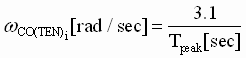(13)

References

 Boulter, B.T., "A Novel Approach for On-Line Self-Tuning Strip Tension Regulation", Proceedings of the 4th IEEE International Conference on Control Applications, pp 91-98, September 1995.

 Boulter, B.T. Fox, H.W.,"Advanced Dynamic Simulation", Reliance Electric Systems Engineering Training Course EO108 1995.

 Carter, W.C., "Reducing Transient Strains in Elastic Processes" , Control Engineering Mar. 1965. pp. 84-87.

 Fox, S.J., Lilley, D.G., "Computer Simulation Of Web Dynamics", Proceedings of the 1st IWHC International Web Handling Conference Tab 20. Oklahoma State University, March 1991.

 Lin, K., "WTS 6.0, A Computer-Based Analysis Program For Multi-Span Web Transport Systems", Oklahoma State University 1994.

 Wolferman, W., "Tension Control of Webs - A Review of the Problems and Solutions in the Present and Future", Tab 15, Proceedings of the 3rd IWHC International Web Handling Conference, Oklahoma State University June 1995.